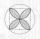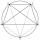# Semicircle

To a semicircle with diameter 10 cm inscribe square. What is the length of square sides?

Result

s =  4.472 cm

#### Solution:Leave us a comment of example and its solution (i.e. if it is still somewhat unclear...):

Showing 0 comments:Be the first to comment!#### To solve this example are needed these knowledge from mathematics:

Looking for help with calculating roots of a quadratic equation? Pythagorean theorem is the base for the right triangle calculator.

## Next similar examples:

1. MO circlesJuro built the ABCD square with a 12 cm side. In this square, he scattered a quarter circle that had a center at point B passing through point A and a semicircle l that had a center at the center of the BC side and passed point B. He would still build a ci
2. QuatrefoilCalculate area of the quatrefoil which is inscribed in a square with side 6 cm.
3. Common chordTwo circles with radius 17 cm and 20 cm are intersect at two points. Its common chord is long 27 cm. What is the distance of the centers of these circles?
4. Eq with reciprocalSolve given equation with reciprocal member: a-6/a+10=4/8
5. The chordCalculate a chord length which the distance from the center of the circle (S, 6 cm) equals 3 cm.
6. Chord 5It is given circle k / S; 5 cm /. Its chord MN is 3 cm away from the center of the circle . Calculate its length.
7. RT and circlesSolve right triangle if the radius of inscribed circle is r=9 and radius of circumscribed circle is R=23.
8. Theorem proveWe want to prove the sentense: If the natural number n is divisible by six, then n is divisible by three. From what assumption we started?
9. FractionFor what x expression ? equals zero?
10. Circle's chordsIn the circle there are two chord length 30 and 34 cm. The shorter one is from the center twice than longer chord. Determine the radius of the circle.
11. Quadratic equationFind the roots of the quadratic equation: 3x2-4x + (-4) = 0.
12. EquationEquation ? has one root x1 = 8. Determine the coefficient b and the second root x2.
13. DiscriminantDetermine the discriminant of the equation: ?
14. RootsDetermine the quadratic equation absolute coefficient q, that the equation has a real double root and the root x calculate: ?
15. Circle chordDetermine the radius of the circle in which the chord 6 cm away from the center of the circle is 12 cm longer than the radius of the circle.
16. 10 piecesHow to divide the circle into 10 parts (geometrically)?
17. Functions f,gFind g(1) if g(x) = 3x - x2 Find f(5) if f(x) = x + 1/2